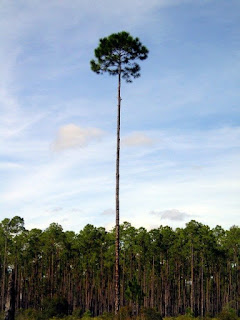## Posts

Showing posts from September, 2019

### How to find the occurrence's of a pattern in a given string Using KMP algorithm?For finding the pattern in a given string , we are going to use KMP algorithm for individual occurrence of the pattern and print the reference index of the original string. The function which will find the pattern in a given string : int KMP(char * str,char* find,int s){ int sl=strlen(str); int fl=strlen(find); for(int i=s;i<sl-fl+1;i++){ int i1=i; for(int j=0;j<fl;j++,i1++){ //cout<<j<<fl<<endl; if(j==fl-1)return i; if(str[i]!=find[j])break; } } return -1; } For finding all the places (positions/index) where the pattern is present, we have to call the above function repetitively with passing the index from where it has to resume the search as a parameter to the function. The same is implemented in the function below: int print_occurence(char* str,char* find){ int sl=strlen(str); int fl=strlen(find); int occ=0; for(int i=0;i<sl;i++){

### How to find the height of binary tree ? program (Python)The height of binary tree is defined as MAX(depth of leaf nodes)  and depth of a node in turn is defined as (n-1) ,where n = the least number of nodes traversed through, to reach that node. For detailed information on Implementing binary tree in python : refer here. Definition of height function: def height(self): if self.root == None: return 0 else: return self._height(self.root, 0) def _height(self, cur_node, cur_height): if cur_node == None: return cur_height left_height = self._height(cur_node.left_child, cur_height + 1) right_height = self._height(cur_node.right_child, cur_height + 1) return max(left_height, right_height) Here, two functions are used one is used to check that if the binary tree exists or not , if exists then it will call the recursive function to traverse the left branch and right branch depth with respect to the root node , and then returns the bigger of the two by comparing. The value of depth(height ) of tre

### How to create a Binary Tree Program ( Python / C / C++ )What is a binary tree? A binary tree is categorized as an abstract data type , whose (I/O) ,(R/W) operations are handled by the user defined functions.A binary tree is a special case of a tree DS where each node except leaf nodes has two children's . for creating a binary tree we will define a class / structure node which will contain the members(left child and right child) and the value. class node: def __init__(self, value=None): self.value = value self.left_child = None self.right_child = None Now , we will write the code for inserting elements in our binary tree, we will create the function in another class containing a member which will store the address of root node and add all successive elements under the root node . def insert(self, value): if self.root == None: self.root = node(value) else: self._insert(value, self.root) def _insert(self, value, cur_node): if value <